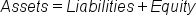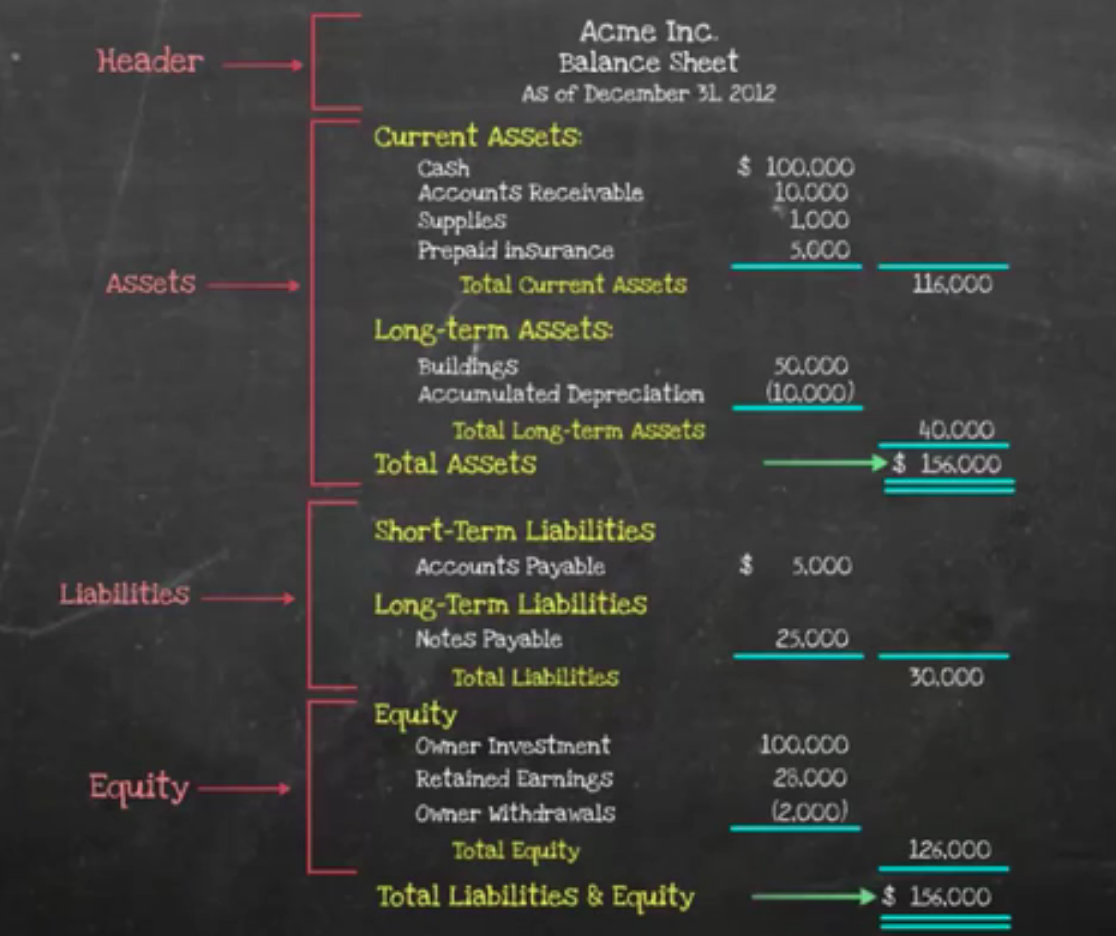+

# Balance Sheet

Author: Sophia Tutorial
##### Description:

Identify the key elements of a balance sheet.

(more)### Developing Effective Teams

*No strings attached. This college course is 100% free and is worth 1 semester credit.

37 Sophia partners guarantee credit transfer.

299 Institutions have accepted or given pre-approval for credit transfer.

* The American Council on Education's College Credit Recommendation Service (ACE Credit®) has evaluated and recommended college credit for 32 of Sophia’s online courses. Many different colleges and universities consider ACE CREDIT recommendations in determining the applicability to their course and degree programs.

Tutorial
what's covered
This tutorial will cover the topic of the balance sheet.

Our discussion breaks down as follows:

1. Balance Sheet
2. Balance Sheet: Example

## 1. Balance Sheet

Before we begin our discussion of the balance sheet, it is important to define financial statements, which are reports providing financial information about a business at a given time. It follows that a balance sheet is a financial statement that provides information about the assets, liabilities, and equity of a business at a given time.

Think of the balance sheet as the business position. It captures a moment in time, rather than spanning a period, and it is the only financial statement that is prepared at a specific date.

hint
The balance sheet is not period-based; it reflects a specific point in time.

The balance sheet details a business's resources, meaning assets, or the resources owned or available, liabilities owed to others, and the net difference on a cumulative basis, which is the equity.

Now, the accounting equation is a fundamental premise in accounting, which states that a company's assets will be equal to the sum of its liabilities and its equity. This also happens to be the balance sheet formula; the accounting equation and the balance sheet formula are the same.

formula
Accounting Equation/Balance Sheet FormulaLet's examine the formula itself in more detail:

• Assets are the physical or non-physical resources owned by an organization that have economic value. These are the available resources.
• Liabilities are the debts and other financial responsibilities of an organization, meaning the monies owed to others, or any obligations of a financial nature.
• Equity is the remaining value once liabilities are subtracted from assets. Equity is the net difference on a cumulative basis.
terms to know
Financial Statements
Balance Sheet
A financial statement that provides information about the assets, liabilities and equity of a business at a given time
Accounting Equation
A fundamental premise in accounting which states that a company's assets will be equal to the sum of its liabilities and its equity (Assets = Liabilities + Equity)
Assets
Physical or non-physical resources owned by an organization that have economic value
Liabilities
Debts and other financial responsibilities of an organization
Equity
The remaining value once liabilities are subtracted from assets

## 2. Balance Sheet: Example

Let's look at an example of a balance sheet, breaking out its component parts:• Header. The header includes the company name, the title "Balance Sheet," and then, because it is at a specific point in time, it has a line that states "As of..."--in this case, "As of December 31, 2012."
• Assets. We start with the current assets--cash, accounts receivable, supplies, and prepaid insurance. Next, we detail the long-term assets, which in this case are the buildings. Both current and long-term assets are added together to provide the total assets.
• Liabilities. The next section comprised liabilities, starting with short-term liabilities--in this case, the accounts payable. Next are the long-term liabilities, which are the notes payable. So, similar to the assets, the liabilities on the balance sheet are broken up into short-term and long-term, and again, we sum the short-term and long-term to arrive at the total liabilities.
• Equity. In this example, the equity items include an owner's investment and retained earnings, which are earnings kept in the business and not distributed to owners. We subtract out the withdrawals to provide the total equity.

Finally, we total up the liabilities and equity, because remember, just like the accounting equation, the balance sheet formula is the same: assets equal liabilities plus equity. Therefore, if we have total assets of \$156,000, you can also see that we have total liabilities and equity of \$156,000--everything balances.

summary
Today we learned about the balance sheet, which is a financial statement that provides information about the assets, liabilities, and equity of a business at a given time. It's important to note that the balance sheet provides information at a specific date. We also learned about the balance sheet formula and the accounting equation: assets equals liabilities plus equity. Lastly, we explored a comprehensive balance sheet example.

Source: Adapted from Sophia instructor Evan McLaughlin.

Terms to Know
Accounting Equation

A fundamental premise in accounting which states that a company's assets will be equal to the sum of its liabilities and its equity (Assets = Liabilities + Equity).

Assets

Physical or non-physical resources owned by an organization that have economic value.

Balance Sheet

A financial statement that provides information about the assets, liabilities and equity of a business at a given time.

Equity

The remaining value once liabilities are subtracted from assets.

Financial Statements